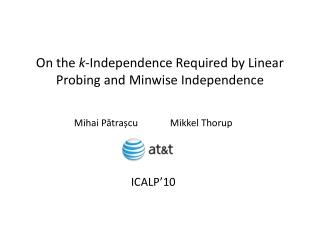Download PresentationOn the k -Independence Required by Linear Probing and Minwise Independence

# On the k -Independence Required by Linear Probing and Minwise Independence - PowerPoint PPT PresentationDownload Presentation## On the k -Independence Required by Linear Probing and Minwise Independence

- - - - - - - - - - - - - - - - - - - - - - - - - - - E N D - - - - - - - - - - - - - - - - - - - - - - - - - - -
##### Presentation Transcript

1. On the k-Independence Required by LinearProbing and Minwise Independence Mihai PătrașcuMikkelThorup ICALP’10

2. Linear Probing h(x) insert(x) [Knuth’63]E[time of one operation] = O(1)“birth of algorithm analysis” • But assumes h is a truly random function ⇒ not an algorithm, but a heuristic

3. Implementable Hash Functions k-independence [Wegman, Carter FOCS’79] As we draw h from a family H: • uniformity: (∀) x∈U, h(x) uniform in [b] • independence: (∀) x1, …, xk∈ U, h(x1), …, h(xk) i.i.d. Possible implementation: • let U = prime field • draw a0, …, ak-1 ∈ U randomly • h(x) = ( ak-1 xk-1 + … + a1x + a0) mod b

4. Understanding Linear Probing [Pagh, Pagh, Ružić STOC’07] 5-independence suffices! Let N(v) = # leafs under v = 2level(v) “Dangerous” if ¾ N(v) elements under it v

5. Understanding Linear Probing Main Lemma: If h(x) is in a run of length 2k⇒ level k-1 ancestor or a sibling must be dangerous One of these must be dangerous h(x) insert(x)

6. Understanding Linear Probing Look at “construction time” = time to insert n elements Main lemma ⇒ construction time ≤ Σdangerous v [N(v)]2 E[construction time] ≤ Σv [N(v)]2 · Pr[v dangerous] 2-independence ⇒Chebyshev bound ⇒ Pr[v dangerous] ≤ 1/N(v)⇒ E[construction time] ≤ Σv N(v) = O(nlgn) Just a classic balls-in-bins analysis!

7. Understanding Linear Probing Look at “construction time” = time to insert n elements Main lemma ⇒ construction time ≤ Σdangerous v [N(v)]2 E[construction time] ≤ Σv [N(v)]2 · Pr[v dangerous] 4-independence ⇒4th moment bound ⇒ Pr[v dangerous] ≤ 1/[N(v)]2⇒ E[construction time] ≤ ΣvO(1) = O(n) Just a classic balls-in-bins analysis!

8. Understanding Linear Probing

9. Understanding Linear Probing One query with k-independence= keys arrange themselves by (k-1)-independence + the query hits a random location

10. Understanding Linear Probing Do we really need “one more” for bounds / operation?

11. Understanding Linear Probing Do we really need “one more” for bounds / operation? YES. Nasty 2-independent family such that: • often, (∃) run of √n elements; • the query often falls in this bad run.

12. Understanding Linear Probing Could 3-independence help?

13. Understanding Linear Probing Could 3-independence help? NO Distribute keys down a tree: If Pr[Case 2] ≈ 1/n ⇒ 3-independence! n keys n/2 0 n/2 n cost Ω (n2) Case 1 Case 2

14. Understanding Linear Probing Can both phenomena hit you simultaneously?

15. Understanding Linear Probing Can both phenomena hit you simultaneously? YES • Apply the bad 3-independent distributiononly on the query path ⇒ 4-independent! • [Nasty proof ]

16. Minwise Independence Problem: how many packets pass through both A and B? Jaccard coefficient: |A ∩ B| / |A ∪ B| Algorithm: • hash and keep min h(A), min h(B) • Pr[min h(A) = min h(B)] = |A ∩ B| / |A ∪ B| • repeat to estimate accurately A B

17. Hashing Guarantees Minwise Independence: for any S, x ∈ S Pr[ h(x) = min h(S) ] = 1 / |S| Implies: Pr[min h(A) = min h(B)] = |A ∩ B| / |A ∪ B|  Minwise independence not easy to obtain

18. Hashing Guarantees ε-Minwise Independence: for any S, x ∈ S Pr[ h(x) = min h(S) ] = (1 ± ε) / |S| Implies: Pr[min h(A) = min h(B)] = (1 ± ε) |A ∩ B| / |A ∪ B| [Indyk SODA’99] Any c·lg(1/ε)-independent family is ε-minwise independent Here: Some c’·lg(1/ε)-independent families are not ε-minwise independent

19. What it All Means All our hash families are artificial… we understand the k-wise independence concept In practice: • (a * x) >> shift • More results: • Ω(nlgn)-construction for linear probing • terrible minwise behavior

20. What it All Means All our hash families are artificial… we understand the k-wise independence concept In practice: • (a * x) >> shift • tabulation-based hashing • Forthcoming paper: • Simple tabulation (3-wise independent) achieves • linear probing in O(1) time (+ Chernoff concentration!) • o(1)-minwise independence

21. What it All Means All our hash families are artificial… we understand the k-wise independence concept In practice: • (a * x) >> shift • tabulation-based hashing The polynomial hash function: • performance not understood • but not too good in practice…

22. The End Open problem: cuckoo hashing6-independence needed [Cohen, Kane]O(lgn)-independence suffices